Test: Power Systems- 9

# Test: Power Systems- 9

Test Description

## 10 Questions MCQ Test GATE Electrical Engineering (EE) 2023 Mock Test Series | Test: Power Systems- 9

Test: Power Systems- 9 for Electrical Engineering (EE) 2023 is part of GATE Electrical Engineering (EE) 2023 Mock Test Series preparation. The Test: Power Systems- 9 questions and answers have been prepared according to the Electrical Engineering (EE) exam syllabus.The Test: Power Systems- 9 MCQs are made for Electrical Engineering (EE) 2023 Exam. Find important definitions, questions, notes, meanings, examples, exercises, MCQs and online tests for Test: Power Systems- 9 below.
Solutions of Test: Power Systems- 9 questions in English are available as part of our GATE Electrical Engineering (EE) 2023 Mock Test Series for Electrical Engineering (EE) & Test: Power Systems- 9 solutions in Hindi for GATE Electrical Engineering (EE) 2023 Mock Test Series course. Download more important topics, notes, lectures and mock test series for Electrical Engineering (EE) Exam by signing up for free. Attempt Test: Power Systems- 9 | 10 questions in 30 minutes | Mock test for Electrical Engineering (EE) preparation | Free important questions MCQ to study GATE Electrical Engineering (EE) 2023 Mock Test Series for Electrical Engineering (EE) Exam | Download free PDF with solutions
 1 Crore+ students have signed up on EduRev. Have you?
Test: Power Systems- 9 - Question 1

### In a 230 kV system, the line to ground capacitance is .03 μF and inductance is 12 mH. If an instantaneous magnetizing current of 8A is interrupted find the output voltage appearing across the pole of a C.B.

Detailed Solution for Test: Power Systems- 9 - Question 1

L = 12 × 10-3 C = .03 × 10-6

Voltage across the breaker poles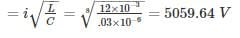*Answer can only contain numeric values
Test: Power Systems- 9 - Question 2

### For an overcurrent relay with plug setting multiplier of 5 and time multiplier setting of 2, the operating time of relay is 5 sec. The operating time when plug setting multiplier is not changed and time multiplier setting is made 0.5 is _____ (in sec)

Detailed Solution for Test: Power Systems- 9 - Question 2

Plug setting multiplier =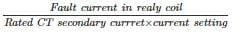Given that for PSM = 5 and TSM = 2,

Operating time = 5 sec.

When PSM = 5, TSM = 0.5

Operating time = 0.5/2 × 5 = 1.25sec

Test: Power Systems- 9 - Question 3

### A 50 Hz, 20 kV generator is connected to a power system. The system reactance and capacitance per phase are: 5 mH and 0.6 mF respectively. What is the maximum voltage across the contacts of the circuit breaker at an instant when it passes through zero

Detailed Solution for Test: Power Systems- 9 - Question 3

Maximum value of restriking voltage

= 2 × peak value of the system voltage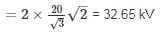*Answer can only contain numeric values
Test: Power Systems- 9 - Question 4

A 10-ampere, over current relay having a current setting of 150% and a time setting multiplier of a 0.8 connected to supply circuit through a 400/5 current transformer when the circuit carries a fault current of 5000 A. Find the plug-setting multiplier.

Detailed Solution for Test: Power Systems- 9 - Question 4

Rated secondary current of current transformer = 5 A

Pickup current = 5 × 1.5 = 7.5 A

Fault current in relay coil =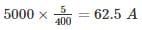Plug-setting multiplier =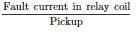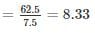Test: Power Systems- 9 - Question 5

A connection of a percentage differential relay is shown in the figure. A high resistance fault occurs near the neutral end with the current distribution the relay has 10% i.e Nr/No = 0.1 and pick up of 0.15 A the CT ratio is 500/5. Find the relay current.

Detailed Solution for Test: Power Systems- 9 - Question 5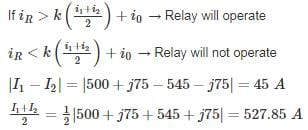Relay operates at 10% = .1 × 527.85 = 52.785 A

Given C.T ratio 500/5

relay current = 52.785/100 = .528A

*Answer can only contain numeric values
Test: Power Systems- 9 - Question 6

A star connected 3 phase, 12 MVA, 8 KV alternator is protected by Merz-Price circulating current principle using 1000/5 amperes current transformers. The start point of the alternator is earthed through a resistance of 10 Ω. If the minimum operating current for the relay is 1 A. Calculate the percentage of each phase of the stator winding which is unprotected against earth-faults when the machine is operating at normal voltage.

Detailed Solution for Test: Power Systems- 9 - Question 6

Let x% of the winding be unprotected.

Earth resistance, r = 10 Ω

Voltage per phase,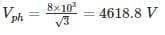Minimum fault current which will operate the relay =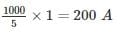EMF induced in x% winding =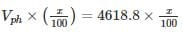= 46.19 x volts

Earth fault current which x% winding will cause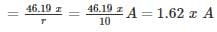⇒ 4.62x = 200

⇒ x = 43.29%

Hence, 43.29% of alternator winding is left unprotected.

*Answer can only contain numeric values
Test: Power Systems- 9 - Question 7

A 50 Hz, 13 kV three phase alternator with earthed neutral has a reactance of 8 ohms per phase and is connected to a bus bar through a circuit breaker. The distributed capacitance upto circuit breaker between phase and neutral is 0.02 μF. The average rate of rise of re-striking voltage up to first peak is (in kv/μsec)

Detailed Solution for Test: Power Systems- 9 - Question 7

Inductance per phase,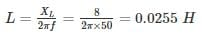Capacitance per phase, C = 0.02 μF

Peak re-striking voltage,=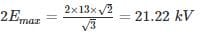Frequency of oscillation,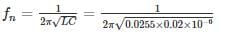= 7047.499 Hz

Peak re-striking voltage occurs at a time given by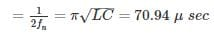Average rate of rise of re-striking voltage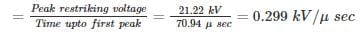*Answer can only contain numeric values
Test: Power Systems- 9 - Question 8

Consider a Δ/Y connected, 20 MVA, 33/11 -kV transformer with differential protection applied for the current transformer ratios shown in figure find the minimum relay current setting to allow 125 percent overload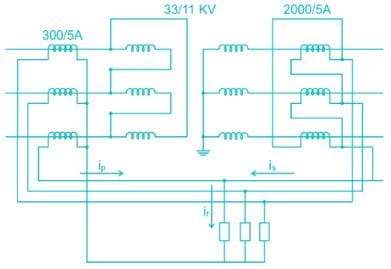Detailed Solution for Test: Power Systems- 9 - Question 8

The primary line current is given by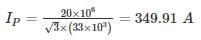The secondary line current is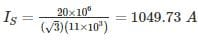The C.T current on the primary side is thus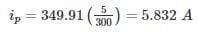The C.T current in the secondary side is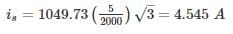Note that we multiply by √3 to obtain the values on the line side of the Δ connected C.T.S. the relay current on Normal load is therefore

ir = ip – is = 5.832 – 4.545 = 1.287 A

with 1.25 overload ratio, the relay setting should be

ir = 1.25 × 1.287 = 1.61A

*Answer can only contain numeric values
Test: Power Systems- 9 - Question 9

A 150 MVA, 22 kV synchronous generator having a reactance of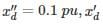= 0.2 pu,xd = 1.1pu is connected to a transformer through a 5-cycle circuit breaker.

The generator is at no load and rated voltage when a three-phase short circuit fault occurs between the breaker and transformer. The ratio of momentary current to sustained short circuit current of breaker is -

Detailed Solution for Test: Power Systems- 9 - Question 9

Momentary current =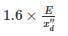Sustained short circuit current =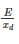The ratio of momentary current to sustained short circuit current of breaker =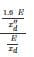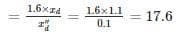Test: Power Systems- 9 - Question 10

A – 3 phase transformer rated for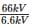is connected star/delta and the protecting current transformer on the low voltage side has a ratio of 500/5. Determine the ratio of the current transformer on the HV side.

Detailed Solution for Test: Power Systems- 9 - Question 10

Since the LT side delta connected, the CTs on that side will be star connected. Therefore, if 500 amps is the line current, the CT secondary current is 5 amps. The line current on the star side of the power transformer will be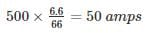The CTs on the star side are delta connected and the current required on the relay side of the CT is 5 amps. Therefore, the current in the CT secondary (Phase Current) is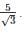The ratio of the current transformer on the HV side is.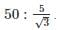## GATE Electrical Engineering (EE) 2023 Mock Test Series

22 docs|274 tests
Information about Test: Power Systems- 9 Page
In this test you can find the Exam questions for Test: Power Systems- 9 solved & explained in the simplest way possible. Besides giving Questions and answers for Test: Power Systems- 9, EduRev gives you an ample number of Online tests for practice

## GATE Electrical Engineering (EE) 2023 Mock Test Series

22 docs|274 tests(Scan QR code)